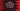# Python program to find all indices of a character in a stringThis python program will show you how to find all indices of a character in a user provided string. Our program will take both the string and character from the user as input. We will learn different ways to find the indices in this post. Let’s take a look at them one by one :

## By using a for loop and if else block :

``````given_str = input("Enter your string : ")
given_char = input("Enter the character to find in the string : ")

for index in range(len(given_str)):
if(given_str[index] == given_char):
print("{} is found in index {}".format(given_char, index))``````

Here, we have two variables : givenstr_ and givenchar. _givenstr_ variable is used to hold the user input string and givenchar_ to hold the user input character.

Using a for loop, we are iterating through the string character indices. Inside the loop, we are checking if the current character is equal to the user input character givenchar_ or not. If both are equal, we are printing a message that the character is found with its index position. **

## By using a while loop :

``````given_str = input("Enter your string : ")
given_char = input("Enter the character to find in the string : ")

index = 0

while(index < len(given_str)):
if(given_str[index] == given_char):
print("{} is found in index {}".format(given_char, index))
index = index + 1``````

We can also solve the same problem by using a while loop as shown above. The while loop runs from index = 0 to index = length of the string - 1. Inside the while loop, we are checking if the current character in the string defined by the index is equal to the user input character or not. If yes, we are printing one message with the index.

## Example programs :

``````Enter your string : hello world
Enter the character to find in the string : o
o is found in index 4
o is found in index 7

Enter your string : ababababababab
Enter the character to find in the string : b
b is found in index 1
b is found in index 3
b is found in index 5
b is found in index 7
b is found in index 9
b is found in index 11
b is found in index 13``````